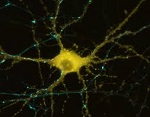# プログラミング ＋ アカデミック + 何か面白いこと

1. Academic
2. 55 view

## Neural Net Learning with Most Decent Method part51. Set initial value to weights and threshold(weight_input_hidden, weight_hidden_output, threshold_hidden), prepare num which is iteration number, δwhich is acceptable difference and learning rate α.
2. Obtain below with input data x^p, p=1,・・・,P.3. Obtain gradients with teacher value d^p, p=1,・・・,P.4. Update parameters using gradient.5. When number of iteration reaches num or square error is lower than δ, stop calculation. If it’s not, go back to step2.